Next: Free precession of Earth Up: Darwin-Radau equation Previous: Derivation of Darwin-Radau equation

# Simple application of Darwin-Radau theory

As a simple application of the preceding analysis, let us assume that the density distribution inside the rotating body is such that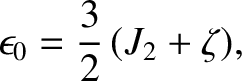(D.54)

for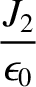, where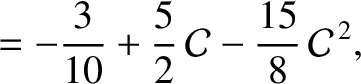. Of course,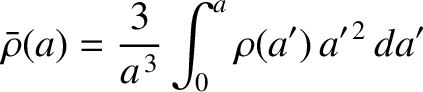for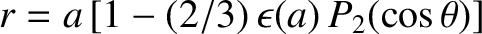. According to Equations (D.26) and (D.42),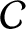(D.55)

where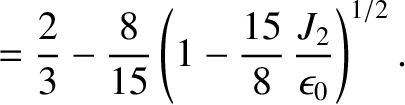(D.56)

Thus, Equation (D.33) yields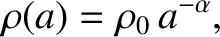(D.57)

Finally, it follows from Equations (D.48), (D.49), and (D.51) that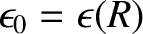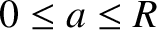(D.58)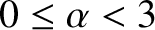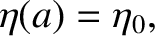(D.59) and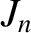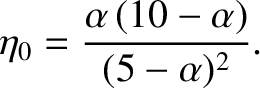(D.60)

respectively.

For the case of the Earth (Yoder 1995),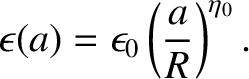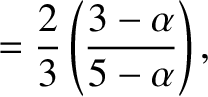(D.61) and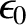(D.62)

These values are consistent with formula (D.59) provided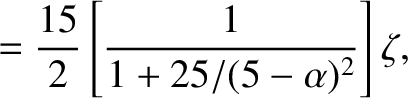, which implies that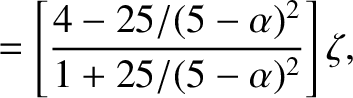. Equations (D.58) and (D.60) then give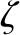(D.63) and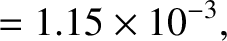(D.64)

respectively. It turns out that these values for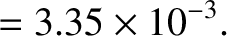and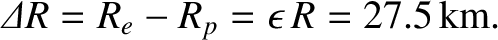are fairly accurate [the true values are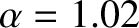and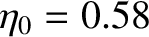(Yoder 1995)]. This suggests that the response of the Earth to its centrifugal potential is essentially fluid-like, and, also, that the Earth's core is significantly denser than its crust.

For the case of Jupiter (Yoder 1995),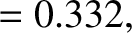(D.65) and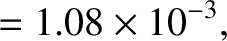(D.66)

These values are consistent with formula (D.59) provided, which implies that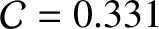. Equations (D.58) and (D.60) then give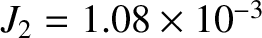(D.67) and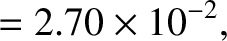(D.68)

respectively. These values forandare somewhat inaccurate [the true values are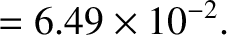and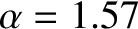(Yoder 1995).] One possible reason for the disagrement is the fact that the values of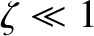and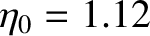are sufficiently large for Jupiter that it is not a good approximation to neglect terms that are second order in these quantities in the analysis (Cook 1980). However, the fact thatfor Jupiter is significantly greater thanfor the Earth suggests that the Jupiter's mass distribution is much more centrally condensed than the Earth's.Next: Free precession of Earth Up: Darwin-Radau equation Previous: Derivation of Darwin-Radau equation
Richard Fitzpatrick 2016-03-31